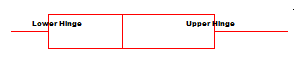# Upper Hinge and Lower Hinge

Descriptive Statistics > Upper Hinge and Lower Hinge

## What are the Upper Hinge and Lower Hinge?

Tukey’s upper hinge and lower hinge are measures of position. The hinges are created when you split a data set into four pieces (with three hinges). As the median is included in this “splitting,” Tukey’s hinges are sometimes called inclusive quartiles.

• The lower hinge is the 25th percentile.
• The midhinge is the 50th percentile (the median).
• The upper hinge is the 75th percentile.

Informally, the lower hinge is equal to the first quartile (Q1) and the upper hinge is equal to the upper quartile (Q3) (See: What is a Quartile?) and the midhinge is equal to the median. The H-spread is the difference Upper Hinge(H2) – Lower Hinge(H1). This is usually equal to the interquartile range. Graphically, the hinges are the bottom end and top end of a box and whiskers chart.Exception to the above: If you divide your sample size by 4, and get a remainder of 3, the hinges will not equal Q1 and Q3. For example, if you had 35 items in your set, then 35/4 = 8 R 3. This is because of Tukey’s formal definition for the upper and lower hinges:

• Lower hinge H1 = an+2 = a(n+3)/4
• Upper hinge H2 = a3n+2 = a(3 n+1)/4

Essentially, if your data set is a multiple of 4, Tukey’s hinges are very easy to calculate. If not, you’ll either have to add some data points (easiest) or do some extrapolation in between numbers (see below for an example with odd numbers). In most cases, when people (professors, authors….) talk about “hinges,” they are referring to Q1 and Q3. But you may want to double check to make sure they aren’t using the formal definition. If they are (and you get that remainder of 3 when dividing by 4), your hinges will not equal the first and third quartiles.

## How to Find the Lower Hinge and Upper Hinge

Need help with a homework question? Check out our tutoring page!

You can think of the lower hinge as the median of the lower half of the data.

## 1. ODD Set of Numbers in the sample.

Example question: Find the lower hinge for the data set 33, 34, 55, 57, 60, 61, 61, 2, 3, 5, 6, 7, 8, 12, 15, 18, 20, 27, 28, 29, 70.
Step 1: Put the numbers in order.
2, 3, 5, 6, 7, 8, 12, 15, 18, 20, 27, 28, 29, 33, 34, 55, 57, 60, 61, 61, 70.
Step 2: Find the median.
2, 3, 5, 6, 7, 8, 12, 15, 18, 20, 27, 28, 29, 33, 34, 55, 57, 60, 61, 61, 70.
Step 3: Place parentheses around the numbers below and including the median.
(2, 3, 5, 6, 7, 8, 12, 15, 18, 20, 27), 28, 29, 33, 34, 55, 57, 60, 61, 61, 70.
Step 4: Find the median (the middle number) of the lower half:
(2, 3, 5, 6, 7, 8, 12, 15, 18, 20, 27), 28, 29, 33, 34, 55, 57, 60, 61, 61, 70.
The lower hinge for this set of data is 8.
Step 5: Find the median (the middle number) of the upper half using the same steps as above:
2, 3, 5, 6, 7, 8, 12, 15, 18, 20, (27, 28, 29, 33, 34, 55, 57, 60, 61, 61, 70).
The upper hinge for this set of data is 55.

## 2. EVEN Set of Numbers in the sample (multiple of 4).

Example question: Find the lower hinge for the data set 33, 34, 55, 57, 60, 61, 61, 3, 5, 6, 7, 8, 12, 15, 18, 20, 27, 28, 29, 70.
Step 1: Put the numbers in order.
3, 5, 6, 7, 8, 12, 15, 18, 20, 27, 28, 29, 33, 34, 55, 57, 60, 61, 61, 70.
Step 2: Find the median point and place a slash mark, cutting the data into two halves.
3, 5, 6, 7, 8, 12, 15, 18, 20, 27,/ 28, 29, 33, 34, 55, 57, 60, 61, 61, 70.
Step 3: Place parentheses around the bottom half of data.
(3, 5, 6, 7, 8, 12, 15, 18, 20, 27)/ 28, 29, 33, 34, 55, 57, 60, 61, 61, 70.
Step 4: Find the median (the middle number) of the lower half:
(3, 5, 6, 7, 8 (10), 12, 15, 18, 20, 27)/ 28, 29, 33, 34, 55, 57, 60, 61, 61, 70.
The lower hinge for this set of data is 10.
The upper hinge, using the same techniques, is 56.

## The Problem With The Median

Caution: different textbooks give slightly different instructions with what to do with the median (e.g. include it or exclude it). That’s because the word “hinge” is sometimes used informally to refer to Q1 or Q3, even though it sometimes differs (if you have a remainder of 3 when dividing by 4). Different calculators also do things differently. If you’re asked to find lower and upper hinges, use your textbook as a guide to avoid getting the “wrong” answer.

References:
Everitt, B. S.; Skrondal, A. (2010), The Cambridge Dictionary of Statistics, Cambridge University Press.
Tukey, J. (1977). Exploratory Data Analysis, Addison-Wesley.

CITE THIS AS:
Stephanie Glen. "Upper Hinge and Lower Hinge" From StatisticsHowTo.com: Elementary Statistics for the rest of us! https://www.statisticshowto.com/upper-hinge-lower-hinge/